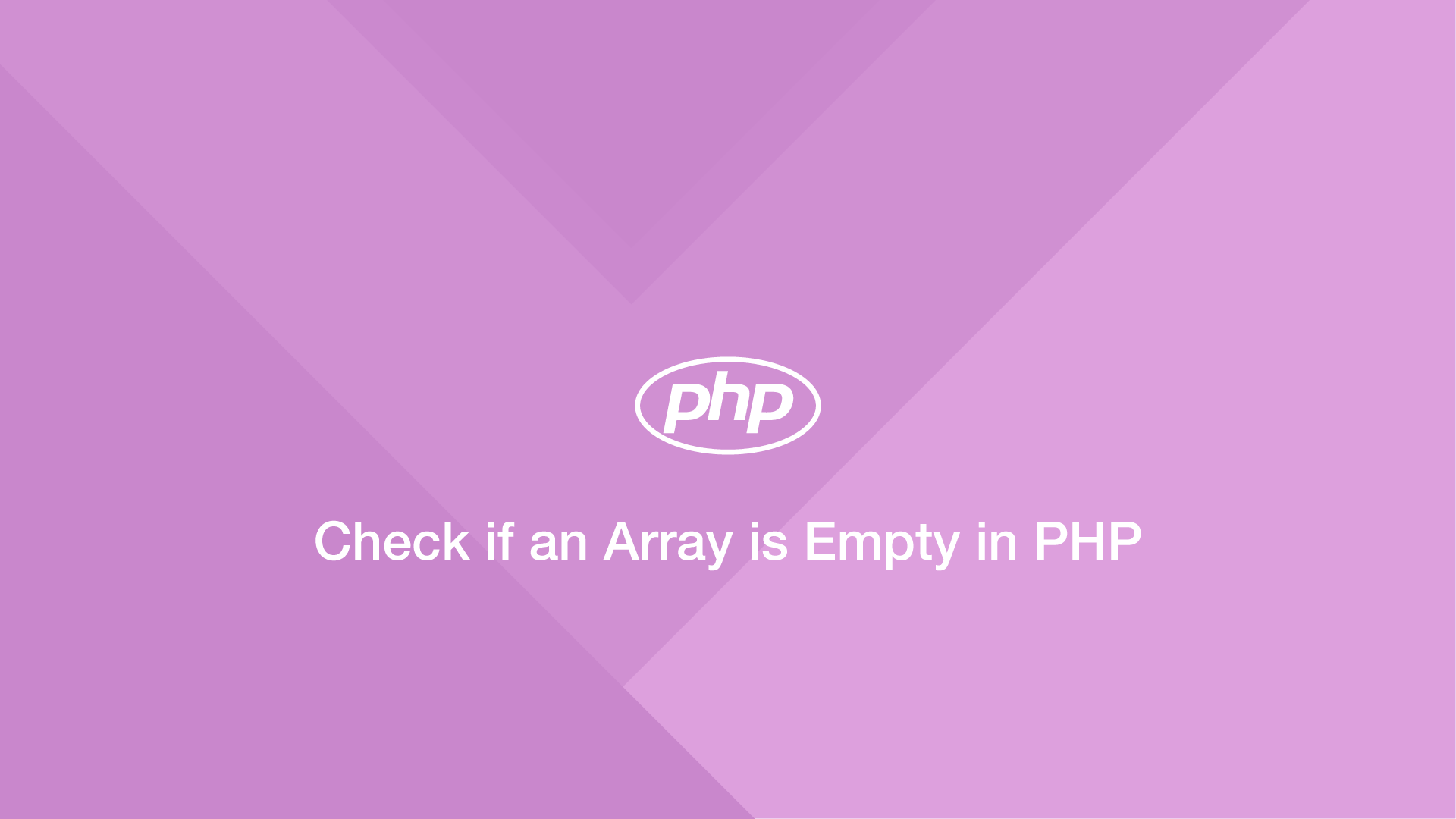# Check if an Array is Empty in PHPAttempting to access an empty array in PHP will probably cause an error to be thrown. It is therefore good practice to check whether an array is empty before using it in your program. There are several ways to go about doing this in PHP, which is what we will learn about in this tutorial.

## Using the empty() Utility

The `empty()` utility was introduced in PHP 4 and is used to check the status of an array. It returns `false` if an array contains elements or the supplied variable is undefined and returns `true` if the array is empty.

``````\$fruit = [];
dd(empty(\$fruit));
``````
``````true
``````
``````\$fruit = ['apple', 'peach', 'orange'];
dd(empty(\$fruit));
``````
``````false
``````

Since `empty()` returns boolean `true`/`false` we can use it in an `if-else` statement to do something if an array variable is defined and contains elements.

``````\$fruit = ['apple', 'peach', 'orange'];

if (!empty(\$fruit)) {
dd('Array is not empty do something');
} else {
dd('Do something else when there is not a populated array');
}
``````

## Using count() to Check if an Array is Empty

The `count()` utility is used to count the number of elements in an array – if there are no elements it will return `0`. If the supplied variable is not an array an error will be thrown, making `count()` only useful if you are certain the variable will always be an array.

``````\$fruit = ['apple', 'peach', 'orange'];

if (count(\$fruit)) {
dd('Array is not empty do something');
} else {
dd('Do something else when there is not a populated array');
}
``````

## Using sizeof()

`sizeof()` can also be used to get the length of an array. If the value returned is `0` the array is empty. Like `count()`, `sizeof()` will throw an error if something other than an array is supplied.

``````\$fruit = ['apple', 'peach', 'orange'];

if (sizeof(\$fruit) == 0) {
dd('Array is not empty do something');
} else {
dd('Do something else when there is not a populated array');
}
``````

## Conclusion

You now know several different ways to check if an array is empty in PHP. `empty()` is the cleanest and most robust option if you don't want any errors being thrown regardless of what data type is supplied.

#### Related Tutorials### How to Create and Use Arrays in JavaScript

July 11, 2020### How to Create a Preview Video Thumbnail of a Video with FFmpeg & PHP

March 23, 2021### How to Add to an Array in PHP

July 02, 2020July 16, 2020